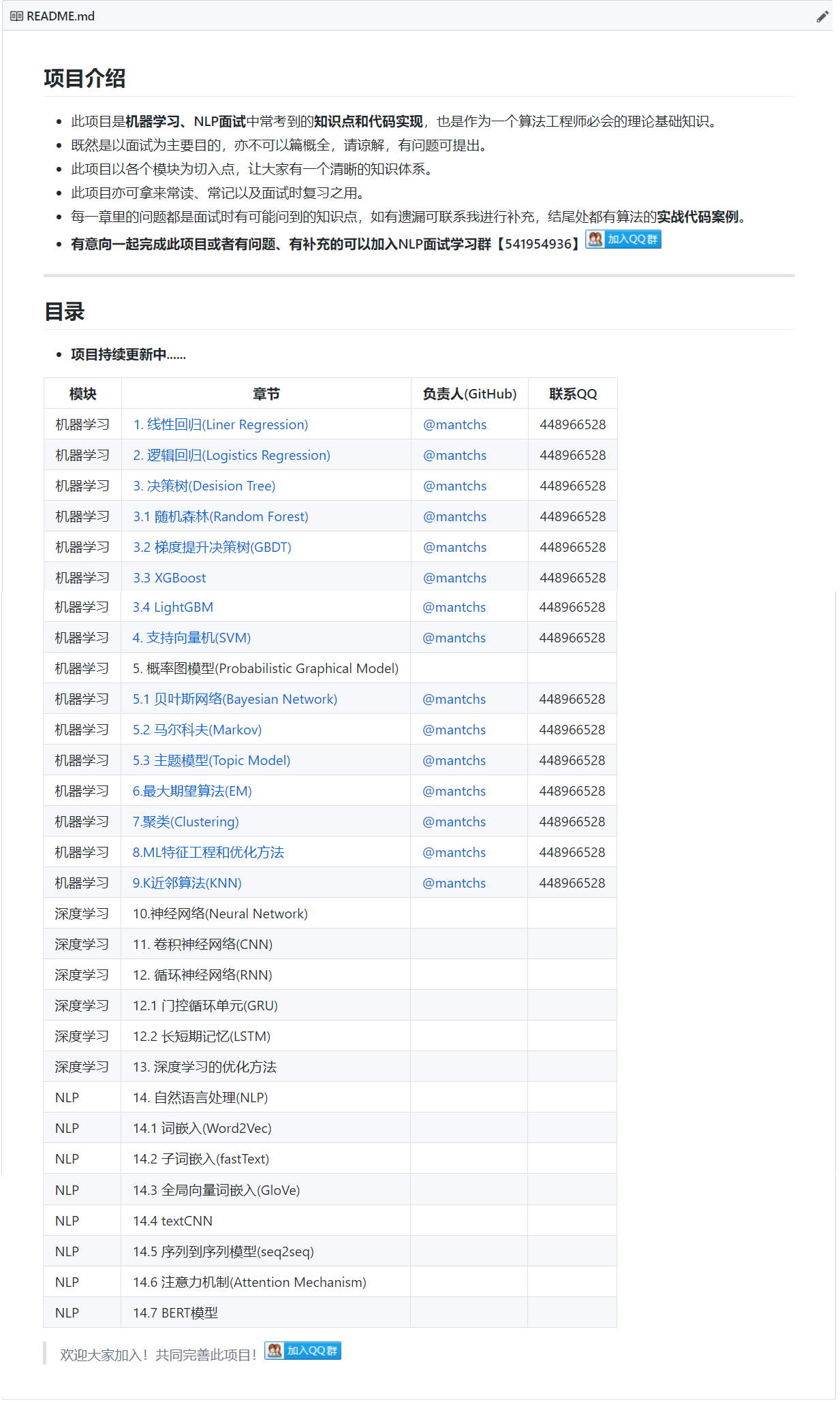# 【深度学习】深度学习-优化方法

## 1. 训练误差和泛化误差

• 训练误差：模型在训练数据集上表现出的误差。
• 泛化误差：模型在任意⼀个测试数据样本上表现出的误差的期望，并常常通过测试数据集上的误差来近似。

## 3. ⽋拟合和过拟合

• 欠拟合：模型⽆法得到较低的训练误差。
• 过拟合：是模型的训练误差远小于它在测试数据集上的误差。

• 如果模型的复杂度过低，很容易出现⽋拟合；
• 如果模型复杂度过⾼，很容易出现过拟合。## 4. 丢弃法(Dropout)

$$h_i=\phi(x_1w_{1i}+x_2w_{2i}+x_3w_{3i}+x_4w_{4i}+b_i)$$

$$h_i^{′}=\frac{\xi_i}{1-p}$$

$$E(h_i^{′})=\frac{E(\xi_i)}{1-p}h_i=h_i$$## 5. 梯度消失/梯度爆炸（Vanishing / Exploding gradients）

1. 预训练加微调

其基本思想是每次训练一层隐节点，训练时将上一层隐节点的输出作为输入，而本层隐节点的输出作为下一层隐节点的输入，此过程就是逐层“预训练”（pre-training）；在预训练完成后，再对整个网络进行“微调”（fine-tunning）。

此方法有一定的好处，但是目前应用的不是很多了。

2. 梯度剪切、正则

梯度剪切这个方案主要是针对梯度爆炸提出的，其思想是设置一个梯度剪切阈值，然后更新梯度的时候，如果梯度超过这个阈值，那么就将其强制限制在这个范围之内。这可以防止梯度爆炸。

另外一种解决梯度爆炸的手段是采用权重正则化（weithts regularization）比较常见的是L1和L2正则。

3. ReLu、leakReLu等激活函数

ReLu：其函数的导数在正数部分是恒等于1，这样在深层网络中，在激活函数部分就不存在导致梯度过大或者过小的问题，缓解了梯度消失或者爆炸。同时也方便计算。当然，其也存在存在一些缺点，例如过滤到了负数部分，导致部分信息的丢失，输出的数据分布不在以0为中心，改变了数据分布。

leakReLu：就是为了解决relu的0区间带来的影响，其数学表达为：leakrelu=max(k*x,0)其中k是leak系数，一般选择0.01或者0.02，或者通过学习而来。

4. Batch Normalization

Batch Normalization是深度学习发展以来提出的最重要的成果之一了，目前已经被广泛的应用到了各大网络中，具有加速网络收敛速度，提升训练稳定性的效果，Batch Normalization本质上是解决反向传播过程中的梯度问题。Batch Normalization，简称BN，即批规范化，通过规范化操作将输出信号x规范化到均值为0，方差为1保证网络的稳定性。

• 有一些从 0 到 1 而不是从 1 到 1000 的特征值，通过归一化所有的输入特征值𝑥，以获得类似范围的值，可以加速学习。所以 Batch 归一化起的作用的原因，直观的一点就是，它在做类似的工作，但不仅仅对于这里的输入值，还有隐藏单元的值。
• 它可以使权重比你的网络更滞后或更深层，比如，第 10 层的权重更能经受得住变化。
5. 残差结构

残差的方式，能使得深层的网络梯度通过跳级连接路径直接返回到浅层部分，使得网络无论多深都能将梯度进行有效的回传。

6. LSTM

LSTM全称是长短期记忆网络（long-short term memory networks），是不那么容易发生梯度消失的，主要原因在于LSTM内部复杂的“门”(gates)。在计算时，将过程中的梯度进行了抵消。

## 6. 随机梯度下降法(SGD)

### 6.1 mini-batch梯度下降

• batch_size=1，就是SGD。
• batch_size=n，就是mini-batch
• batch_size=m，就是batch

• batch：相对噪声低些，幅度也大一些，你可以继续找最小值。
• SGD：大部分时候你向着全局最小值靠近，有时候你会远离最小值，因为那个样本恰好给你指的方向不对，因此随机梯度下降法是有很多噪声的，平均来看，它最终会靠近最小值，不过有时候也会方向错误，因为随机梯度下降法永远不会收敛，而是会一直在最小值附近波动。一次性只处理了一个训练样本，这样效率过于低下。
• mini-batch：实践中最好选择不大不小的 mini-batch，得到了大量向量化，效率高，收敛快。

### 6.2 调节 Batch_Size 对训练效果影响到底如何？

1. Batch_Size 太小，模型表现效果极其糟糕(error飙升)。
2. 随着 Batch_Size 增大，处理相同数据量的速度越快。
3. 随着 Batch_Size 增大，达到相同精度所需要的 epoch 数量越来越多。
4. 由于上述两种因素的矛盾， Batch_Size 增大到某个时候，达到时间上的最优。
5. 由于最终收敛精度会陷入不同的局部极值，因此 Batch_Size 增大到某些时候，达到最终收敛精度上的最优。

## 7. 优化算法

### 7.1 动量法$$v_t\leftarrow\gamma_{}v_{t-1}+\eta_tg_t$$

$$x_t\leftarrow_{}x_{t-1}-v_t$$### 7.2 AdaGrad算法

AdaGrad算法会使⽤⼀个小批量随机梯度gt按元素平⽅的累加变量st。在时间步0，AdaGrad将s0中每个元素初始化为0。在时间步t，⾸先将小批量随机梯度gt按元素平⽅后累加到变量st：

$$s_t=s_{t-1}+g_t⊙g_t$$

$$x_t=x_{t-1}-\frac{\eta}{\sqrt{s_t+\varepsilon}}⊙g_t$$

• 如果⽬标函数有关⾃变量中某个元素的偏导数⼀直都较⼤，那么该元素的学习率将下降较快；
• 反之，如果⽬标函数有关⾃变量中某个元素的偏导数⼀直都较小，那么该元素的学习率将下降较慢。### 7.3 RMSProp算法

$$s_t=\gamma_{}s_{t-1}+(1-\gamma)g_t⊙g_t$$

$$x_t=x_{t-1}-\frac{\eta}{\sqrt{s_t+\varepsilon}}⊙g_t$$### 7.4 AdaDelta算法

AdaDelta算法也像RMSProp算法⼀样，使⽤了小批量随机梯度gt按元素平⽅的指数加权移动平均变量st。在时间步0，它的所有元素被初始化为0。给定超参数0 ≤ ρ < 1（对应RMSProp算法中的γ），在时间步t > 0，同RMSProp算法⼀样计算：

$$s_t=ps_{t-1}+(1-p)g_t⊙g_t$$

$$g_t^{′}=\sqrt{\frac{\Delta{}x_{t-1}+\varepsilon}{s_t+\varepsilon}}⊙g_t$$

$$\Delta{}x_t=p\Delta{}x_{t-1}+(1-p)g_t^{′}⊙g_t^{′}$$

### 7.5 Adam算法

Adam算法在RMSProp算法基础上对小批量随机梯度也做了指数加权移动平均。

Adam算法使⽤了动量变量vt和RMSProp算法中小批量随机梯度按元素平⽅的指数加权移动平均变量st，并在时间步0将它们中每个元素初始化为0。给定超参数0 ≤ β1 < 1（算法作者建议设为0.9），时间步t的动量变量vt即小批量随机梯度gt的指数加权移动平均：

$$v_t=\beta_1v_{t-1}+(1-\beta_1)g_t$$

$$s_t=\beta_{2}s_{t-1}+(1-\beta_2)g_t⊙g_t$$

$$\check{v}_t=\frac{v_t}{1-\beta_1^t}$$

$$\check{s}_t=\frac{s_t}{1-\beta_2^t}$$

$$g_t^{′}=\frac{\eta{}\check{v}_t}{\sqrt{\check{s}_t}+\varepsilon}$$

$$x_t=x_{t-1}-g_t^{′}$$

### 7.6 局部最优–鞍点问题## 8. 如何解决训练样本少的问题

1. 利用预训练模型进行迁移微调（fine-tuning），预训练模型通常在特征上拥有很好的语义表达。此时，只需将模型在小数据集上进行微调就能取得不错的效果。CV有ImageNet，NLP有BERT等。
2. 数据集进行下采样操作，使得符合数据同分布。
3. 数据集增强、正则或者半监督学习等方式来解决小样本数据集的训练问题。

## 9. 如何提升模型的稳定性？

1. 正则化（L2, L1, dropout）：模型方差大，很可能来自于过拟合。正则化能有效的降低模型的复杂度，增加对更多分布的适应性。
2. 提前停止训练：提前停止是指模型在验证集上取得不错的性能时停止训练。这种方式本质和正则化是一个道理，能减少方差的同时增加的偏差。目的为了平衡训练集和未知数据之间在模型的表现差异。
3. 扩充训练集：正则化通过控制模型复杂度，来增加更多样本的适应性。
4. 特征选择：过高的特征维度会使模型过拟合，减少特征维度和正则一样可能会处理好方差问题，但是同时会增大偏差。

## 10. 有哪些改善模型的思路

1. *数据角度 *

增强数据集。无论是有监督还是无监督学习，数据永远是最重要的驱动力。更多的类型数据对良好的模型能带来更好的稳定性和对未知数据的可预见性。对模型来说，“看到过的总比没看到的更具有判别的信心”。

2. 模型角度

模型的容限能力决定着模型可优化的空间。在数据量充足的前提下，对同类型的模型，增大模型规模来提升容限无疑是最直接和有效的手段。

3. 调参优化角度

如果你知道模型的性能为什么不再提高了，那已经向提升性能跨出了一大步。 超参数调整本身是一个比较大的问题。一般可以包含模型初始化的配置，优化算法的选取、学习率的策略以及如何配置正则和损失函数等等。

4. 训练角度

在越大规模的数据集或者模型上，诚然一个好的优化算法总能加速收敛。但你在未探索到模型的上限之前，永远不知道训练多久算训练完成。所以在改善模型上充分训练永远是最必要的过程。充分训练的含义不仅仅只是增大训练轮数。有效的学习率衰减和正则同样是充分训练中非常必要的手段。

## 11. 如何提高深度学习系统的性能

1. 提高模型的结构。
2. 改进模型的初始化方式，保证早期梯度具有某些有益的性质，或者具备大量的稀疏性，或者利用线性代数原理的优势。
3. 择更强大的学习算法。

## 12. 参考文献